# PHY3: WORK, ENERGY AND POWER

##### This unit is about work, power and machines and how they are measured.

WORK, ENERGY AND POWER

Energy

This is defined as the capacity to do work. The units for energy are Joules [J]

Chemical energy stored in coal, gas and oil can be released inform of heat when burned e.g. the food we eat has chemical energy in it. This energy is released by chemical reaction so that the body can utilize it.

Electricity is also another form of energy

The sun provides light or radiant energy

Nuclear energy is released by nuclear reaction

Potential energy

This is the energy a body has by virtue its position. A body raised to some height has potential energy. A wound up string has potential energy and a stretched classic bund has potential energy

Kinetic energy

This is the energy a body possesses by virtue of its motion.

WORK

This is said to be done when the point where force is applied moves in the direction of the force

Work = force x distance moved in the direction of force the s.1 unit of work is the joule

Definition of joule

The joule is the work done when the point of application of a force of 1N moves through 1m in the direction of the force.

Interchange of energy between potential and kinetic energy

As a pendulum oscillates its energy can be either kinetic energy or potential energy or both.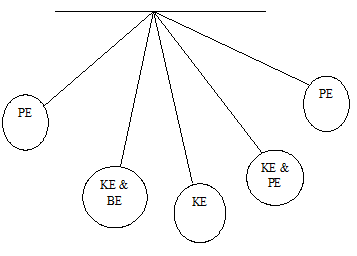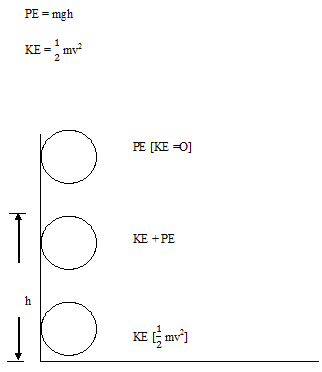When the object has not yet been released it has potential energy and as it falls, it loses its potential energy and gains kinetic energy. Just as the object is about to touch the ground all its potential energy is converted to kinetic energy

The law of conservation

It states that energy cannot be created or destroyed but can be changed from one form to another e.g. potential energy to kinetic energy, electrical to heat energy.[ in iron and cookers] light energy to chemical energy in plants.

POWER

This is the rate at which work is done or it is the rate of transfer of energy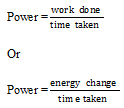The s.1 unit is a watt or joules/ sec

Definition of a watt

The watt is the rate of working of one joule per second i.e. 1 watt of power is used up when one joule of energy is used up per second

SOURCE OF ENERGY

• Wind energy

Wind mills transfer the energy of wind into mechanical energy which can be used to pump water or produce electricity

• Electric energy

This is energy due to an electric current which flows in a circuit

• Chemical energy

This refers to the heat obtained from fuel such as wood, cool, oil, gas etc. it is used to generate other forms of energy, heat from chemical to light

• Solar energy

Concave mirrors collect sunrays and focus them on to special steam boilets which provide the mechanical force to run generators.

• Nuclear energy

This is energy released by nuclear reactions for example a bomb explosion

• Bio- thermal energy

This is obtained from cow dung that is organic plant and animal wastes.

• Geothermal energy

This is obtained from that hot springs and volcanoes. Energy from volcanoes can be used for creating electricity and that from hot springs can be used for boiling eggs and many other things.

MACHINES

This is any device that uses a force applied at one point to overcome another r force applied at another point.

The force applied to the machine is called effort and the force it over comes is load [ie the effort is the force applied at one point to overcome the load]

The s.1 unit for effort /load is the Newton [ N] example of machines include;-

• Pulley
• Bicycles
• Wheel barrows
• Hammer
• Lever
• Screws etc
• Pair of scissors
• Indeed planes
• Gears
• Wheel and axle.

Machines simply work by one or more of the following ways;-

1. Transforming energy for example a generator mechanic
2. Energy into electrical energy
• Ii transferring energy from one place to another for example in a motor car. Energy is transferred from a chunk shaft to drive shaft so as to move the wheels.
1. Multiplying a force e.g. in pulleys
2. Multiplies speed in bicycles

The 3 basic quantities used in relation to machines are the following

Mechanical advantage [MA]. This is ratio of load to effort and has no units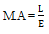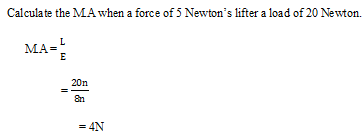Velocity ratio [V.R] is the ratio of the distance mode by effort to the distance moved by load during the same time interview.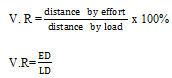Efficiency of a machine [J]. This is the ratio of the useful output of a machine to the work input expressed as percentage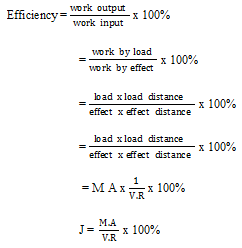Examples of machines

Lever

This is a device of [machine] that consists of a rigid body pivoted at a point called full Crum. [It is any device which can turn about a fulcrum]

Classes of levers

There are 3 classes of lever. They are the 1st, 2nsd and 3rd.

• 1st class lever

These were the fulcrum is found between the load and the effort e.g. the crowbarX and y are perpendicular distance of the load and effect from the pivot respectively. Taking moments about a fulcrum;-

Load x load distance = effort x effort distance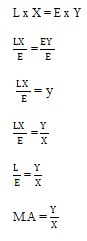Note.

The M.A is equal to V.R.

Other examples of 1st class levers are: claw hammer, see – saw pair of scissors, spanner, and bottle opener etc.

• 2nd class lever

These were the load is in between the fulcrum and the effort e.g. wheel barrow and nutcracker.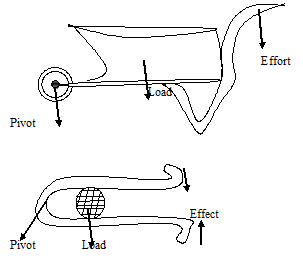• 3rd class lever

This is where the effort is in between the pivot and the load e.g. tongs, tweezers.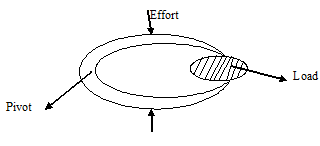Pulleys

A pulley is a wheel with a grooved rim. Pulleys are used in lifting heavy loads to higher positions. They are also used in cranes to off load heavy cargo from ships and in dock yards.A single fixed pulley is used to raise small loads to the top of a building during construction. When we neglect the weight of the rope and a friction, the load is therefore equal to the one. Since the distances moved by the effort and load are the same then V.R = 1. Therefore efficiency is 100%.

Maximum mechanical advantage implies that we obtain greater convenience and ease of being able to stand on the ground and pull the rope down wards, instead of pulling upwards from the top of the building

Note:

The velocity ration is equal to the number of pulleys used or no of strings supporting the load.

Assumptions

1. – The grove of the wheel is assumed to be smooth.
2. – The wheel is light [ or weightless]
3. – The rope used is weightless and is inextensible.

SINGLE MOVABLE PULLEY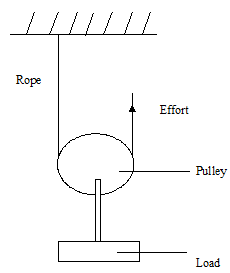Neglecting the weight of the rope and friction, the tension in the rope is equal to the effort. The total upward force on the pulley is therefore twice the effort [E] since the load is supported by 2 ropes. The total tension of the two section of the rope is equal to 2 E thus load is 2E.

The M.A = 2 also

• R = 2 since the number of ropes that support the load is 2.

BLOCK AND TACKLE SYSTEM

A block is the name given to two or more pulleys that are filled on the same frame.

A block and tackle consists of two sections of pulleys that form the upper and lower blocks. This arrangement of pulleys is used to achieve a higher velocity ratio and mechanical advantages. The upper blocks are fixed while the lower blocks are movable.

If the number of pulleys is odd, the string is attached or tied to the lower movable block, however if the number is attacked to the upper fixed blocks.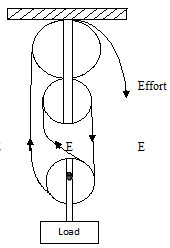AN EXPERIMENT ILLUSTRATES FLOW A4. A AND EFFICIENCY OF PULLEY VARY WITH THE LOADV.R = 4

M.A = 4

An initial small mass is added to the load pan and further are then added to the effort pan until the load just rises slowly with a steady velocity. The experiment is repeated with different loads and the results are recorded in a table as shown below.

 Load [N] Effect [N] M.A V.R EFFICIENCY

GRAPHS OF MECHANICAL ADVANTAGES AGAINST LOAD AND EFFICIENCY AGAINST LOAD ARE THEY PLOTTED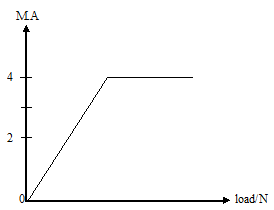M.A increases with the load until value of 4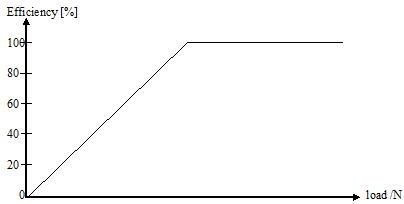Efficiency increases with the load until 100%

Note

Weight of the string depends on the distance between the pulley and the blocks but the weight of the lower remains constant.

The friction varies with load and becomes smaller as the load increases i.e. when the load is small work done due to the weight of the movable parts, a ropes/strings and friction is proportionally high.

But as the total load increases the work done to overcome friction and load becomes smaller and so mechanical advantage increases. The efficiency also increases with the load for the same reasons.

The efficiency of any machine e.g. pulley is never 100 efficient because;-

1. There is work wasted in overcoming friction
2. More work is wasted in raising the movable parts of the machine.

A block and tackle has three pulleys in the upper fixed block and 2 in the lower movable rock.

What load can be lifted by an effort of 200N, if the efficiency of the system is 60%?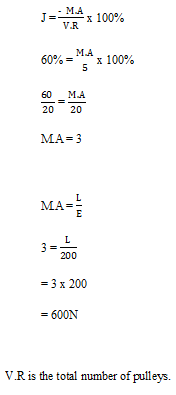This consists of a wheel to which an effort is applied and an axle to which the load is applied. The wheel and axle both have the same axis of rotation for a complete revolution, distance moved by an effort is equal to the circumference of a wheel while the distance moved by the loadAdvantages of the wheel and axle

A small force is applied at the wheel to overcome a large load placed at the axle.

Wheel and axle systems are widely used in gear boxes

Gears

These are commonly found in clocks, watches and cars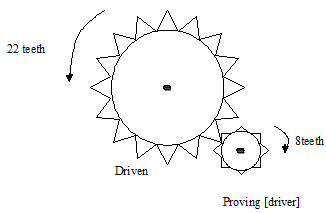The gear system consists of two wheels or more. That turns in opposite direction. They carry projection called teeth that inter log and courses the opposite motion. The wheel that carries the effort is called the driving gear while the wheel that carries the load is called the driven greater. The V.R.O this system depends on the number of teeth on these gears and is defined as follows.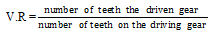Examples

Two gear wheels A and B with 80 and 20 teeth respectively, lock into each other. They are fastened on axles of equal diameters; such that a weight of 150N attached to a string wound round the first axle raises a load of 450N attached to a string wound round the second axle as shown below.

Calculate;-

1. Velocity ratio
2. Efficiency of a system.

A – 80 teeth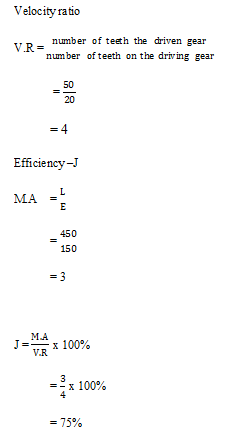Inclined plane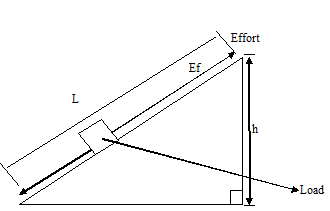An inclined plane is used for raising a heavy load through some height more conveniently as compared to lifting the load, vertically upward. If the length of the inclined is L and the height through which the load is raises is h. Then;-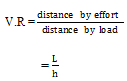M.A is determined by using the principle of conservation of energy thus if the inclined plane is smooth, then work done on load is equal to work done on the effort.

Load x load distance = effort x effort distance

L x h                =          E X L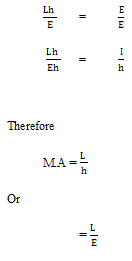M.A = V.R is true if the plane is a perfect included plane.

Other machines

That includes; the screw/car jerk, the screw driver, the borer, the G. glides, the clump e.t.c

Have a section that contains threads which acts on the load and a rod on which the effort is applied.

When the effort traverses a distance equal to circumference swept by the rod. Then the load move through a distance equal between any two threads. This distance and this distance are called the pitch.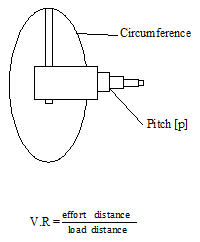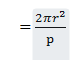THIS VIDEO EXPLAINS MORE ABOUT WORK, POWER AND ENERGY.

## STEM Elearning

We at FAWE have built this platform to aid learners, trainers and mentors get practical help with content, an interactive platform and tools to power their teaching and learning of STEM subjects, more

#### How to find your voice as a woman in Africa

© FAWE, Powered by: Yaaka DN.